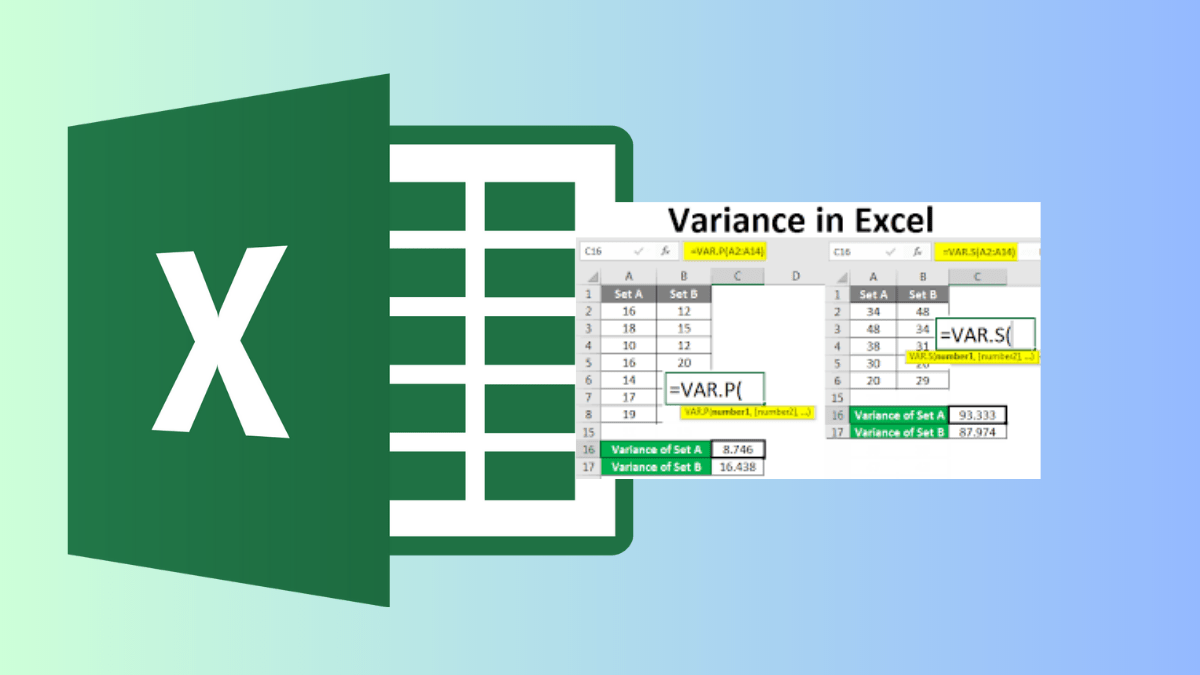# How to Calculate Variance in ExcelVariance is a statistical measure that helps us understand how spread out a set of data points is from their mean (average) value. In simpler terms, it indicates the extent to which individual data points deviate from the average. Excel, with its powerful data analysis capabilities, makes calculating variance a breeze. In this guide, we will walk you through the process of calculating variance in Excel using easy-to-understand steps and real-world examples.

Contents

## Understanding Variance

Before we dive into the Excel calculations, let’s grasp the concept of variance. Imagine you’re tracking the weekly earnings of a grocery shop. You want to know how much the daily earnings deviate from the average weekly earnings. Variance helps you quantify this deviation, shedding light on the data’s volatility.

Example Data: Weekly Earnings of a Coffee Shop

READ ALSO: How to Use Quick Analysis Tool in Excel

### Calculating Variance using Excel’s Built-in Function

Step 1: Enter the Data

Enter the data set into an Excel spreadsheet.

Step 2: Use Excel’s Variance Function

In Excel, you can use the VAR.P function to calculate the population variance or the VAR.S function to calculate the sample variance. Since we’re working with a sample data set, let’s use VAR.S.

In an empty cell, type the following formula:

=VAR.S(B2:B6)

Here, B2:B6 represents the range of cells containing your data.

Press Enter, and Excel will calculate the sample variance.

For our example data set:

=VAR.S(500, 550, 600, 480, 520)

The calculated sample variance using Excel’s function is approximately 2200.

Interpreting the Result

In our grocery shop example, the calculated variance is approximately 2200. This value indicates the average squared deviation of weekly earnings from their mean. A higher variance suggests greater variability in earnings from week to week.

### Conclusion

Calculating variance in Excel is a vital statistical tool that helps you understand the distribution and volatility of data. With simple formulas and built-in functions, you can quickly perform these calculations, enabling you to make informed decisions based on data variability. Whether you’re analyzing business earnings or scientific experiments, mastering variance calculation in Excel equips you with the insights needed to navigate the world of data with confidence.